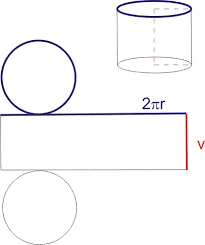# Calculation 81405

Sketch the mesh of a cylinder whose base radius to height ratio is 2 : 3. Calculate the volume and surface of the cylinder if its height is 9 cm (sketch, calculation, answer).

V =  1017.876 cm3
S =  565.4867 cm2

### Step-by-step explanation:Did you find an error or inaccuracy? Feel free to write us. Thank you!

Tips for related online calculators
Need help calculating sum, simplifying, or multiplying fractions? Try our fraction calculator.
Check out our ratio calculator.